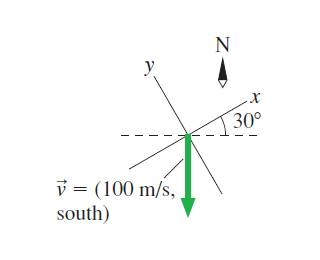# What are the x- and y-components of the velocity vector?

## Homework Statement

Question is: What are the x- and y-components of the velocity vector shown in the figure?https://session.masteringphysics.com/problemAsset/1384003/6/knight_Figure_03_22.jpg

## The Attempt at a Solution

I tried -100 m/s*cos(30 degrees)=-86.6m/s for the x component and -100m/s*sin(30 degrees)=-50m/s for the y component, but was incorrect for both. What am I missing?

berkeman
Mentor

## Homework Statement

Question is: What are the x- and y-components of the velocity vector shown in the figure?https://session.masteringphysics.com/problemAsset/1384003/6/knight_Figure_03_22.jpg

## The Attempt at a Solution

I tried -100 m/s*cos(30 degrees)=-86.6m/s for the x component and -100m/s*sin(30 degrees)=-50m/s for the y component, but was incorrect for both. What am I missing?

Welcome to the PF.

You got the sin & cos backwards. Try drawing the line from the tip of the vector to the negative y axis. See how the component of the vector on the negative y axis involves the cos(30 degrees)?

Thanks berkeman, I see what you mean now. I didn't expect something like that. Thank you for the incredibly quick reply.

•berkeman